Warning: Illegal string offset 'html' in /home/hsn/public_html/forum/cache/skin_cache/cacheid_1/skin_topic.php on line 909

Writing maths in your posts with LaTeX - HSN forum# Writing maths in your posts with LaTeX

3 replies to this topic

### #1Nathan

Fully Fledged Genius

•• Members
•• 1,736 posts
• Location:Aberdeen, Scotland
• Gender:Male

Posted 22 March 2007 - 04:39 AM

Looking through the Maths forum last night (yes, see, I do do other things on here rather than just spam up the "What's on your mind" thread), I noticed that there's loads of posts that are misleading as they've not been explained properly (see my first example below).

Hopefully people actually read this and take heed, just so that I haven't wasted 30 mins of my valuable time.PS. Point out anything that I've missed out/got wrong. Thanks, Nathan----------------------------------------------------------

LaTeX is a special type of coding which can help you and others when posting for help on the HSN Maths forums. Basically, it stops confusion when it comes to things like:

x2/5x+3

I mean, is this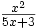,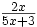, or something entirely different?

It's really simple to use once you get the hang of it, and for the basics, you only need to remember a few simple words that'll get you writing equations in no time at all.

Writing in LaTeX

To write in LaTeX in this forum, you need to put the LaTeX in [tex ] and [/tex ] tags. For example,

CODE
$$x^2$$

becomes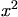Plus/Minus

To use the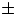, use

CODE
$$\pm$$

Multiplying and Dividing

For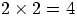, you would use

CODE
$$2 \times 2= 4$$

For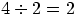, you would use

CODE
$$4 \div 2 = 2$$

Fractions

Example: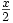would be
CODE
$$\frac{x}{2}$$

Note the backslash (\) and use of curly brackets ({&}). If you miss out the \ or use the wrong type of brackets, then the code won't work!

Multiple lines of working

You can put more than 1 line of working in [tex ] tags. All you need to do is put a double backslash (\\) at the start of each new line.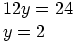CODE
$$\\12y=24 \\y=2$$

Calculus

For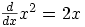, use

CODE
$$\frac{d}{dx}x^2=2x$$

For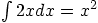, use
CODE
$$\int 2x dx = x^2$$

Greek Letterswould be

CODE
$$\pi \theta \delta \alpha$$

Fractions

To get larger fractions, either use "display" tags rather than "tex" ones, e.g., or use "\dfrac", e.g.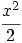Alligning equations

The "display" one is more powerful, since that lets you do multiple lines of equations aligned at the = sign, e.g.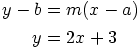CODE
[display]
y-b &=m(x-a) \\
y &= 2x + 3
[/display]

The "&=" is used instead of just "=", since the & symbol tells latex where you want things to line up.

The only other thing I would point out is trig - if you just do "sin x" it looks funny -. You should really do "\sin x", with the slash, so that the "sin" bit appears upright:.

This also applies to "\cos x" and "\tan x".

Logs

Also, for logs: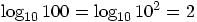CODE
$$\log_{10}100=\log_{10}10^2=2$$

Edited by Nathan, 08 April 2007 - 11:43 AM.

### #2The Wedge Effect

HSN Legend

•• Members
•• 2,477 posts
• Location:Paisley
• Gender:Male

Posted 07 April 2007 - 10:42 PM

Why hasn't this been pinned yet?### #3George

Child Prodigy

••• 720 posts
• Location:West Lothian
• Gender:Male

Posted 08 April 2007 - 11:29 AM

DoneAnd Nathan - to get larger fractions, either use "display" tags rather than "tex" ones, e.g., or use "\dfrac", e.g.The "display" one is more powerful, since that lets you do multiple lines of equations aligned at the = sign, e.g.CODE
[display]
y-b &=m(x-a) \\
y &= 2x + 3
[/display]

The "&=" is used instead of just "=", since the & symbol tells latex where you want things to line up.

The only other thing I would point out is trig - if you just do "sin x" it looks funny -. You should really do "\sin x", with the slash, so that the "sin" bit appears upright:.

This also applies to "\cos x" and "\tan x". Also, for logs:CODE
$$\log_{10}100=\log_{10}10^2=2$$

### #4thedealer07

• Gender:Female

Posted 16 May 2007 - 04:21 PM

mental: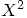#### 1 user(s) are reading this topic

0 members, 1 guests, 0 anonymous users